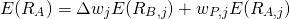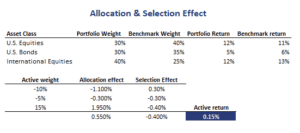# Allocation Effect

The allocation effect and security selection effect are two components of an active strategy’s return. We can easily decompose the sources of return using this simple method. This helps us to identify a manager’s sources of active return. We illustrate the return decomposition method using an Excel example. The template can be downloaded at the bottom of this page.

## Asset allocation vs stock selection

Before we continue, let’s first go over the intuition behind the allocation effect and the security selection effect. A manager can outperform the benchmark in two ways. First, the manager can overweight certain asset classes which subsequently outperform and underweight asset classes that subsequently underperform. The return resulting from this over-weighting and under-weighting is the allocation effect. Alternatively, the manager can keep the weights of the asset classes constant. Instead, he or she could select individual securities within the asset classes that are expected to outperform. A positive return from this is the security selection return.

## Allocation effect and Stock Selection effect

Given the above distinction, we can decompose active return into two components, the asset allocation return and the stock selection return. The asset allocation return is the result of deviations from the asset class portfolio weights of the benchmark. The security selection return results from deviations from benchmark weights within asset classes.

## Allocation effect formula

The formula we need to use to decompose active returns is the followingwhere the weights are active weights. Note that we use both the returns of the benchmark (denoted with B) and the returns of the active portfolio (denoted with P). The first component in the above formula is the return that results from asset allocation. The second component is the return from security selection.

## Return decomposition example

The best way to grasp the methodology, is by looking at an actual example. The following table implements the approach using an example with three asset classes; US equities, US bonds, and International stocks.## Summary

We discussed the asset allocation effect. We showed that the allocation effect is an important component of managers’ active return. The other important component is security selection.

### Allocation effect calculator

Want to have an implementation in Excel? Download the Excel file: Allocation Effect calculator